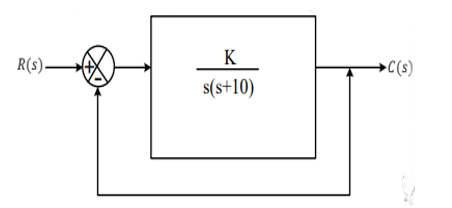Engineering Jobs   »   ELECTRICAL GATE QUIZ

# GATE’22 EE: Daily Practices Quiz 11-Aug-2021

Each question carries 2 marks
Negative marking: 2/3 mark

Total Questions: 05
Total marks: 10
Time: 12 min.

Q1. The unity feedback system shown in the figure has:(c) Steady-state position error K/10 units

Q2. The magnitudes of the open–circuit and short–circuit input impedance of a transmission line are 100 Ω and 25 Ω respectively. The characteristic impedance of the line is.
(a) 25 Ω
(b) 50 Ω
(c) 75 Ω
(d)100 Ω

Q3. The number FF in hexadecimal system has equivalence in decimal system to
(a) 256
(b) 255
(c) 240
(d) 239

Q4. A three-phase 440V, 6 poles, 50Hz, squirrel cage induction motor is running at a slip of 5%. The speed of stator magnetic field w.r.t rotor magnetic field and speed of rotor with respect to stator magnetic field are
(a) Zero, -50 rpm
(b) Zero, 955 rpm
(c) 1000 rpm, -50 rpm
(d) 1000 rpm, 955 rpm

Q5. For a single phase, two winding transformers, the supply frequency and voltage are both increased by 10%. The percentage changes in the hysteresis loss and eddy current loss, respectively, are
(a) – 10 and 21
(b) 21 and 10
(c) – 21 and 10
(d) 10 and 21

SOLUTIONS
S1. Ans.(a)
Sol. G(s)=K/s(s+10) ;H(s)=1
∵position error constant=k_p=lim┬(s→0)⁡〖G(s)H(s)=(lim⁡)┬(s→0) 〗 K/s(s+10) ×1=ꝏ

S2. Ans.(b)
Sol. Z_0=√(Z_OC×Z_SC )=√(100×25)=50 Ω.

S3. Ans.(b)
Sol. (FF)_16=15×16^1+15×16^0=240+15=255.

S4. Ans.(a)
Sol. N_S=(120 f)/P=(120*50)/6=1000 rpm
Rotor speed=N_s-sN_S=950 rpm
Stator magnetic field speed=N_S=1000 rpm
Rotor magnetic field speed=N_S=1000 rpm
So, the relative speed between stator magnetic field and rotor magnetic field=1000-1000=zero
Rotor speed w.r.t stator magnetic field=950-1000=-50 rpm

S5. Ans.(d)
Sol. for a single-phase transformer, both V and f are increased by 10%. Hence, the ratio of (V/f) is constant.
∴W_h α f and W_e α f^2
So, hysteresis loss will be increased by 10 % and eddy current loss will be increased by (1.21-1) =21%.

Sharing is caring!